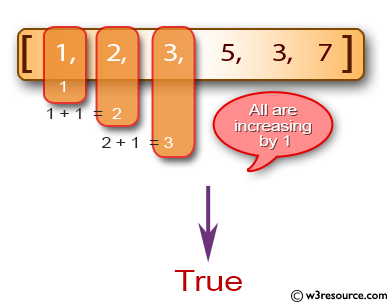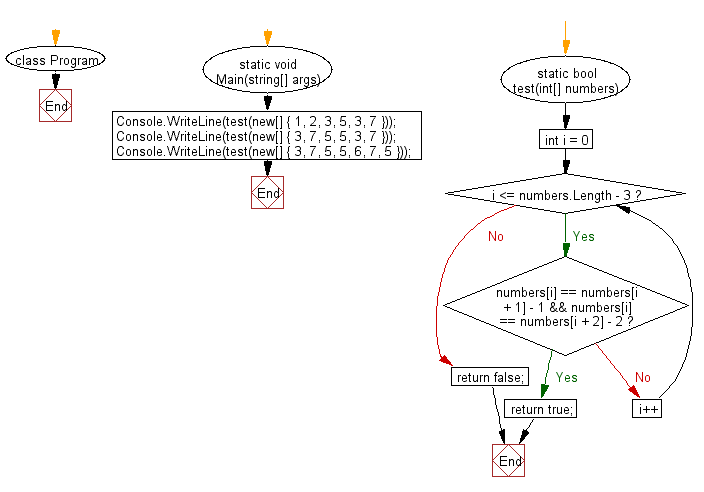﻿ C# - Check an array contains 3 increasing adjacent numbers# C# Sharp Basic Algorithm Exercises: Check a given array of integers and return true if the array contains three increasing adjacent numbers

## C# Sharp Basic Algorithm: Exercise-126 with Solution

Write a C# Sharp program to check a given array of integers and return true if the array contains three increasing adjacent numbers.

Pictorial Presentation:Sample Solution:

C# Sharp Code:

``````using System;
namespace exercises
{
class Program
{
static void Main(string[] args)
{
Console.WriteLine(test(new[] { 1, 2, 3, 5, 3, 7 }));
Console.WriteLine(test(new[] { 3, 7, 5, 5, 3, 7 }));
Console.WriteLine(test(new[] { 3, 7, 5, 5, 6, 7, 5 }));
}
static bool test(int[] numbers)
{
for (int i = 0; i <= numbers.Length - 3; i++)
{
if (numbers[i] == numbers[i + 1] - 1
&& numbers[i] == numbers[i + 2] - 2)
{
return true;
}
}
return false;
}
}
}
```
```

Sample Output:

```True
False
True```

Flowchart:C# Sharp Code Editor:

Improve this sample solution and post your code through Disqus

What is the difficulty level of this exercise?

Test your Programming skills with w3resource's quiz.

﻿